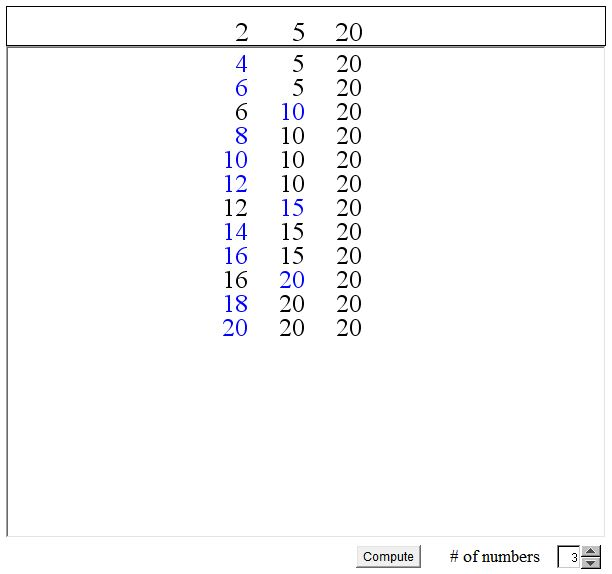# Algorithm for Computing the LCM

The applet below illustrates an algorithm for finding the Least Common Multiple (LCM) of a number of integers.

Let there be a finite sequence of positive integers X = (x1, x2, ..., xn), n > 1. The algorithm proceeds in steps as follows: on each step m it examines and updates the sequence X(m) = (x1(m), x2(m), ..., xn(m)), X(1) = X. The purpose of the examination is to pick the least (perhaps, one of many) element of the sequence X(m). Assuming xk0(m) is the selected element, the sequence X(m+1) is defined as

xk(m+1) = xk(m), k ≠ k0

xk0(m+1) = xk0(m) + xk0.

In other words, the least element is increased by the corresponding x whereas the rest of the elements pass from X(m) to X(m+1) unchanged.

The algorithm stops when all elements in sequence X(m) are equal. Their common value L is exactly LCM(X).

(In the applet the numbers x1, x2, ..., xn that appear at the top row are modifiable by clicking left or right off their vertical center line. Press button Compute to see the algorithm running. The blue numbers the ones that have been modified at one of the steps.)

### If you are reading this, your browser is not set to run Java applets. Try IE11 or Safari and declare the site https:///www.cut-the-knot.org as trusted in the Java setup.What if applet does not run?

DiscussionLet Mk = max xi(k). There are only finitely many k such that Mk ≤ L. Let m be the largest such index. I.e., we assume that Mm ≤ L whereas Mm+1 > L provided the algorithm does not stop at step m. This assumption leads to a contradiction.

Indeed, by a suitable re-numbering, we will then have

 X(m) = (r·x1, x2(m), ..., xn(m)) and X(m+1) = ((r+1)·x1, x2(m), ..., xn(m)),

with r a positive integer and

L ≠ r·x1 ≤ x2(m) ≤ ... ≤ xn(m) = Mm ≤ L

Now (r+l)·x1 ≤ L would imply that Mm+1 ≤ L which could not be, by the maximality of m. Hence

r·x1 < L < (r+1)·x1.

But this contradicts the fact that L is a multiple of x1. Hence the assumption that m is not the last step is false and

X(m)= (Mm, Mm, ..., Mm).

But then Mm is a common multiple of all the xi, i = 1, 2, ..., n, and L ≥ Mm, at that. However, since L is the least common multiple, L ≤ Mm. Hence

L = Mm

and

X(m)= (L, L, ..., L),

as required.

### Remark

This algorithm could be used to simulate a multitasking environment. In fact it was so used in the early days of computing. Assume, for example, you write a program which shows several balls bouncing up and down and off the walls. A task is associated with each ball that regularly updates its position and velocity. All balls must be continuously controlled, i.e. all the associated tasks need to be executed on a rotating basis. In addition, some balls may have to be updated more frequently than others.

The solution is this: each task k is assigned the (reversed) relative frequency xk and the LCM algorithm is run. One step of task k is executed whenever xk(m) is updated. The above discussion shows that all tasks will be updated with the required frequencies.

It's not hard to imagine why the algorithm is thought to resemble a patch of growing onions which are cut individually when they reach a prescribed height. Indeed in networking there is an important Onion Routing algorithm. In computational geometry, a similar idea led to the Onion Peeling Algorithm.

### Reference

1. A. J. Goldman; N. J. Fine, El655, The American Mathematical Monthly, Vol. 71, No. 9. (Nov., 1964), pp. 1045-1046.• Factoring with the Factor Tree
• GCD and LCM via Factor Tree
• GCD and LCM by Plain Factorization
• Common Multiples and the Least Common Multiple
• GCD(M, N) x LCM(M, N) = M x N
• Divisibility Criteria
• Euclid's Algorithm
• Algorithm for Computing the LCM
• Factors And Multiples
• Two Properties of Greatest Common Divisor
• Properties of GCD and LCM
• A Line in a Square Grid
• Number of Factors of an Integer
• Extension of Euclid's Game
•